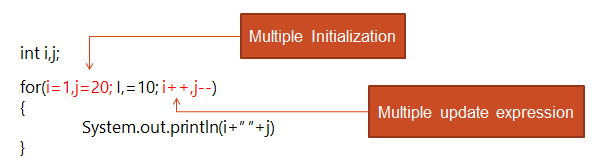` Variations in for Loop`

A for loop can have multiple variations that makes more flexible and efficient.

• Multiple initialization and update expression
• Optional expression
• Infinite loop
• Empty loop

Multiple initialization and update expression

A for loop may contain multiple initialization and update expressions. The multiple expressions must be separated by commas.Optional Expression

By now you are familiar that a for loop contains three types of expressions: initialization expression(condition) and an update expression. But, all these expressions are optional, i.e., we can skip any or all of these expressions depending upon the requirement of the program.

Case 1:

`int i=2;for( ;i<=20;i=i+2){    System.out.println(i);}`

Case 2:

`int i=2;for( ;i<=20; ){    System.out.println(i);    i=i+2;}`

Case 3:

`int i=2;for( ; ; ){  System.out.println(i);   i=i+2;   if(i>20)   {	break;  }}`

Infinite Loop

A for loop will execute its statement block infinite number of times if we skip the condition. If the test expression (condition) is not present in the loop, it will never return false. Hence, it will execute endlessly and become an infinite loop.

Case 1

`int Ifor(i=1; ;i++){     System.out.pritln(i);}`

Case 2

`int i;for( ; ; ){   System.out.pritln(i);}`

Case 3

`int i;for(i=1;i<=i;i++){   System.out.pritln(i);}`

Here , i<=I will always be true.

Empty Loop

A loop without any statement in its body is called an empty loop

`int ifor(i=2;i<=50;i++){}`

Or

`for(i=2;i<=50;i++);`